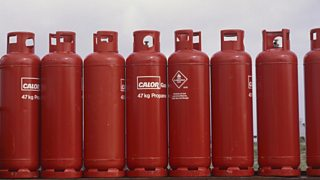Energy from fuels

Combustion reactions

Many different compounds can be used as fuels, most commonly alkanes and alcohols. When these fuels burn, they release heat energy and light energy to the surroundings in exothermic reactions. Endothermic reactions take in heat energy.

These reactions are known as combustion reactions and can be represented by different equations.For example, propane gas tanks are commonly sold on petrol station forecourts. These gas bottles have a wide variety of uses including portable heating and cooking for caravans and barbecues.

When propane burns in a plentiful supply of oxygen, it produces both carbon dioxide and water. This change can be represented by a word equation or a formula equation.

Word equation

$Propane + oxygen \to carbon\,dioxide + water$

Balanced formulae equation

${C_3}{H_8} + 5{O_2} \to 3C{O_2} + 4{H_2}O$

A balanced formulae equation, along with the formula triangles you learned in Unit one can be used to calculate information on the quantities of reactants or products involved in a particular reaction.

Fuels are compounds that react with oxygen to release energy